# NCERT Solutions class-11 Maths Exercise 1.1## myCBSEguide App

CBSE, NCERT, JEE Main, NEET-UG, NDA, Exam Papers, Question Bank, NCERT Solutions, Exemplars, Revision Notes, Free Videos, MCQ Tests & more.

Exercise 1.1

(i) The collection of all the months of a year beginning with the letter J.

(ii) The collection of ten most talented writers of India.

(iii) A team of eleven best-Cricket batmen of the world.

(iv) The collection of all boys in your class.

(v) The collection of all natural numbers less than 100.

(vi) A collection of novels written by the writer Munshi Prem Chand.

(vii) The collection of all even integers.

(viii) The collection of questions in the chapter.

(ix) A collection of most dangerous animals of the world.

Ans. (i) The collection of all months of a year beginning with J is {January, June, July}, which is well defined and hence it forms a set.

(ii) The collection of most talented writers of India is not well defined because opinions about ‘most talented writers’ vary from person to person and hence it does not form a set.

(iii) A team of eleven best-cricket batmen of the world us not well defined because opinion about ‘best-cricket batsmen’ vary from person to person and hence it does not form a set.

(iv) The collection of all boys in your class is well defined and hence it forms a set.

(v) The collection of all natural numbers less than 100 is {1, 2, 3, ……., 99} which is well defined and hence it forms a set.

(vi) A collection of novels written by the writer Munshi Prem Chand is well defined and hence it forms a set.

(vii) The collection al all even integers is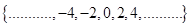which is well defined and hence it forms a set.

(viii) The collection of questions in this chapter is well defined and hence it forms a set.

(ix) A collection of most dangerous animals of the world is not well defined because opinion about ‘most dangerous animals’ vary from person to person and hence it does not form a set.

2. Let A = {1, 2, 3, 4, 5, 6}. Insert the appropriate symbol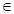or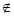on the blank space:

(i) 5 _______ A

(ii) 8 _______ A

(iii) 0 _______ A

(iv) 4 _______ A

(v) 2 _______ A

(vi) 10 _______ A

Ans. Given: A = {1, 2, 3, 4, 5, 6}

(i) 5 is an element of set A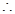5A

(ii) 8 is not an element of set A8A

(iii) 0 is not an element of set A0A

(iv) 4 is an element of set A4A

(v) 2 is an element of set A2A

(vi) 10 is not an element of set A10A

3. Write the following sets in roster form:

(i) A = {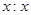is an integer and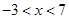}

(ii) B = {is a natural number less than 6}

(iii) C = {is a two-digit natural number such that the sum of its digits is 8}

(iv) D = {is a prime number which is divisor of 60}

(v) E = The set of all letters in the word TRIGONOMETRY

(vi) F = The set of all letters in the word BETTER

Ans. (i) A = {is an integer and}A =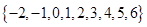(ii) B = {is a natural number less than 6}B = {1, 2, 3, 4 5}

(iii) C = {is a two-digit natural number such that the sum of its digits is 8}C = {17, 26, 35, 44, 53, 62, 71, 80}

(iv) D = {is a prime number which is divisor of 60}D = {2, 3, 5}

(v) E = The set of all letters in the word TRIGONOMETRYE = {T, R, I, G, O, N, O, M, E, T, R, Y}

(vi) F = The set of all letters in the word BETTERF = {B, E, T, R}

4. Write the following sets in the set-builder form:

(i) {3, 6, 9, 12}

(ii) {2, 4, 8, 16, 32}

(iii) {5, 25, 125, 625}

(iv) {2, 4, 6, ……}

(v) {1, 4, 9, ………, 100}

Ans. (i) Let A = {3, 6, 9, 12}.

Here all objects of the set are natural numbers that are multiples of 3.A =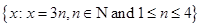(ii) Let B = {2, 4, 8, 16, 32}

Here all objects of the set are natural numbers that are power of 2.B =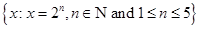(iii) Let C = {5, 25, 125, 625}

Here all objects of the set are natural numbers that are power of 5.C =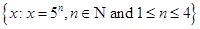(iv) Let D = {2, 4, 6, ……}

Here all objects of the set are even natural numbers.D =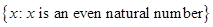(v) Let E = {1, 4, 9, ………, 100}

Here all objects of the set are perfect square.D =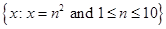5. List all the elements of the following sets:

(i) A = {is an odd natural number}

(ii) B = {is an integer,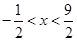}

(iii) C = {is an integer,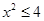}

(iv) D = {is a letter in the word “LOYAL”}

(v) E = {is a month of a year not having 31 days}

(vi) F = {is a consonant in the English alphabet which precedes K}

Ans. (i) A = {is an odd natural number}A = {1, 3, 5, 7, ……..}

(ii) B = {is an integer,}B = {0, 1, 2, 3, 4}

(iii) C = {is an integer,}C =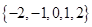(iv) D = {is a letter in the word “LOYAL”}D = {L, O, Y, A}

(v) E = {is a month of a year not having 31 days}E = {February, April, June, September, November}

(vi) F = {is a consonant in the English alphabet which precedes K}F = {B, C, D, F, G, H, J}

6. Match each of the set on the left in the roster form with the same set on the right described in the set-builder form:

(i) {1, 2, 3, 6}

(a) {is a prime number and a divisor of 6}

(ii) {2, 3}

(b) {is an odd natural number less than 10}

(iii) {M, A, T, H, E, I, C, S}

(c) {is a natural number and divisor of 6}

(iv) {!, 3, 5, 7, 9}

(d) {is a letter of word “MATHEMATICS”}

Ans. The sets which are in set-builder form can be written as

(a) {is a prime number and a divisor of 6} = {2, 3}

(b) {is an odd natural number less than 10} = {1, 3, 5, 7, 9}

(c) {is a natural number and divisor of 6} = {1, 2, 3, 6}

(d) {is a letter of word “MATHEMATICS”} = {M, A, T, H, E, I, C, S}

Hence the correct matching is:

(i)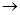(c)

(ii)(a)

(iii)(d)

(iv)(b)